# RD Sharma Solutions For Class 12 Maths Exercise 19.21 Chapter 19 Indefinite Integrals

RD Sharma Solutions for Class 12 Maths Exercise 19.21 Chapter 19 Indefinite Integrals is provided here. The solutions are explained in understandable language, which improves grasping abilities among students. The set of experts at BYJU’S conduct vast research on each topic before designing the solutions, as per prescribed syllabus of the CBSE board.

For more knowledge about the concepts covered in this exercise, students can refer to RD Sharma Solutions Class 12 Maths Chapter 19 Indefinite Integrals Exercise 19.21 PDF, which is available here. This exercise explains the evaluation of integrals of the form

$$\begin{array}{l}\int \frac{px+q}{\sqrt{ax^{2}+bx+c}}\ dx\end{array}$$
by substitution method.

## Download the PDF of RD Sharma Solutions For Class 12 Chapter 19 – Indefinite Integrals Exercise 19.21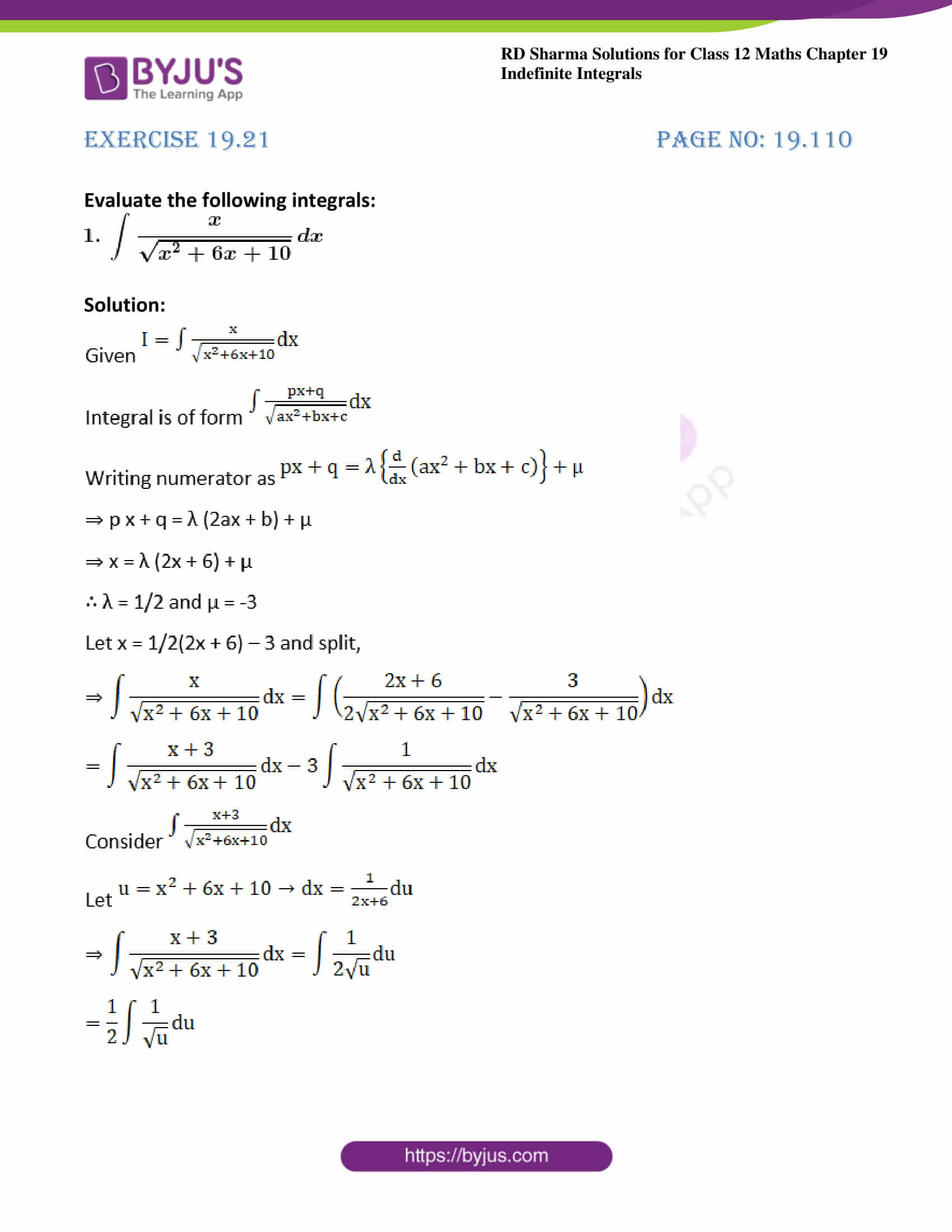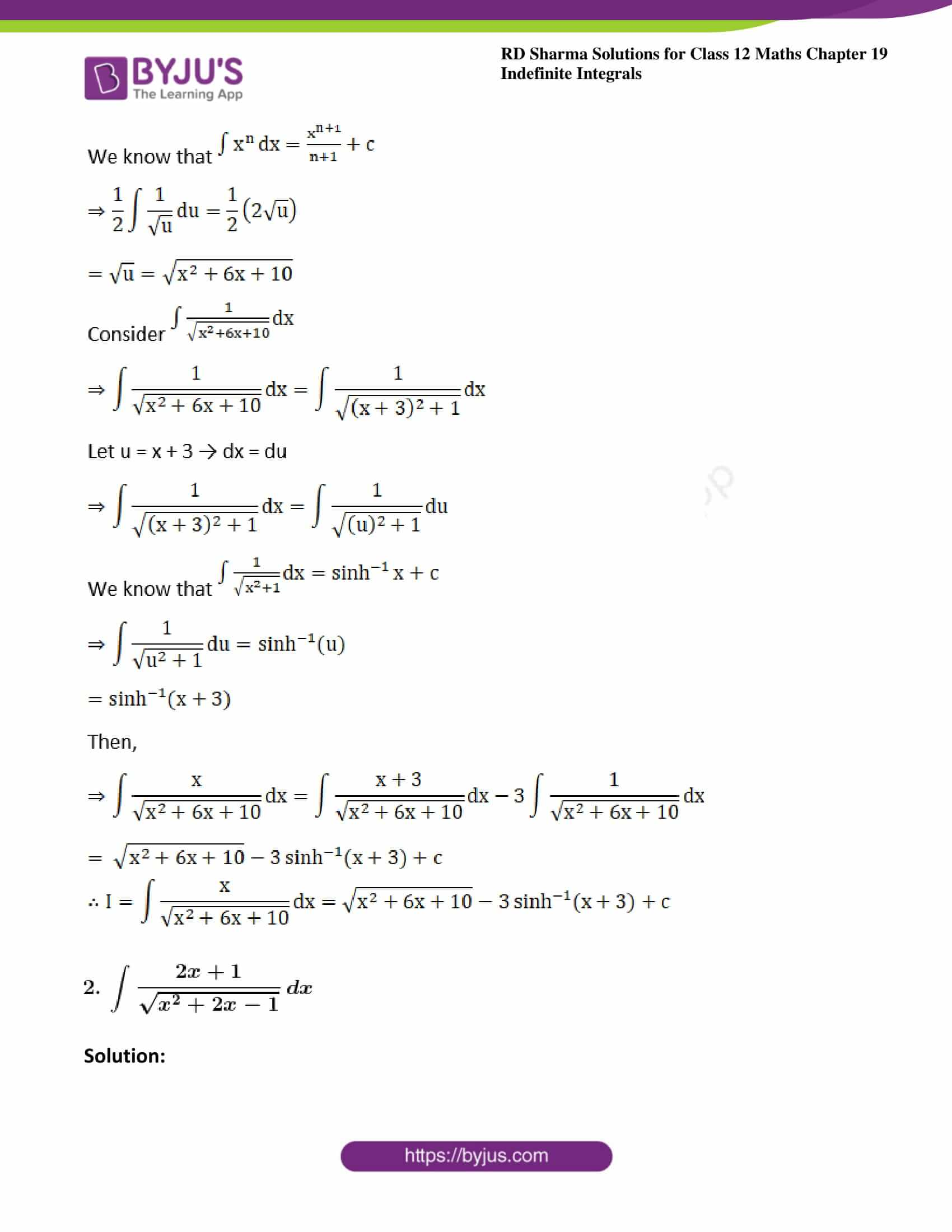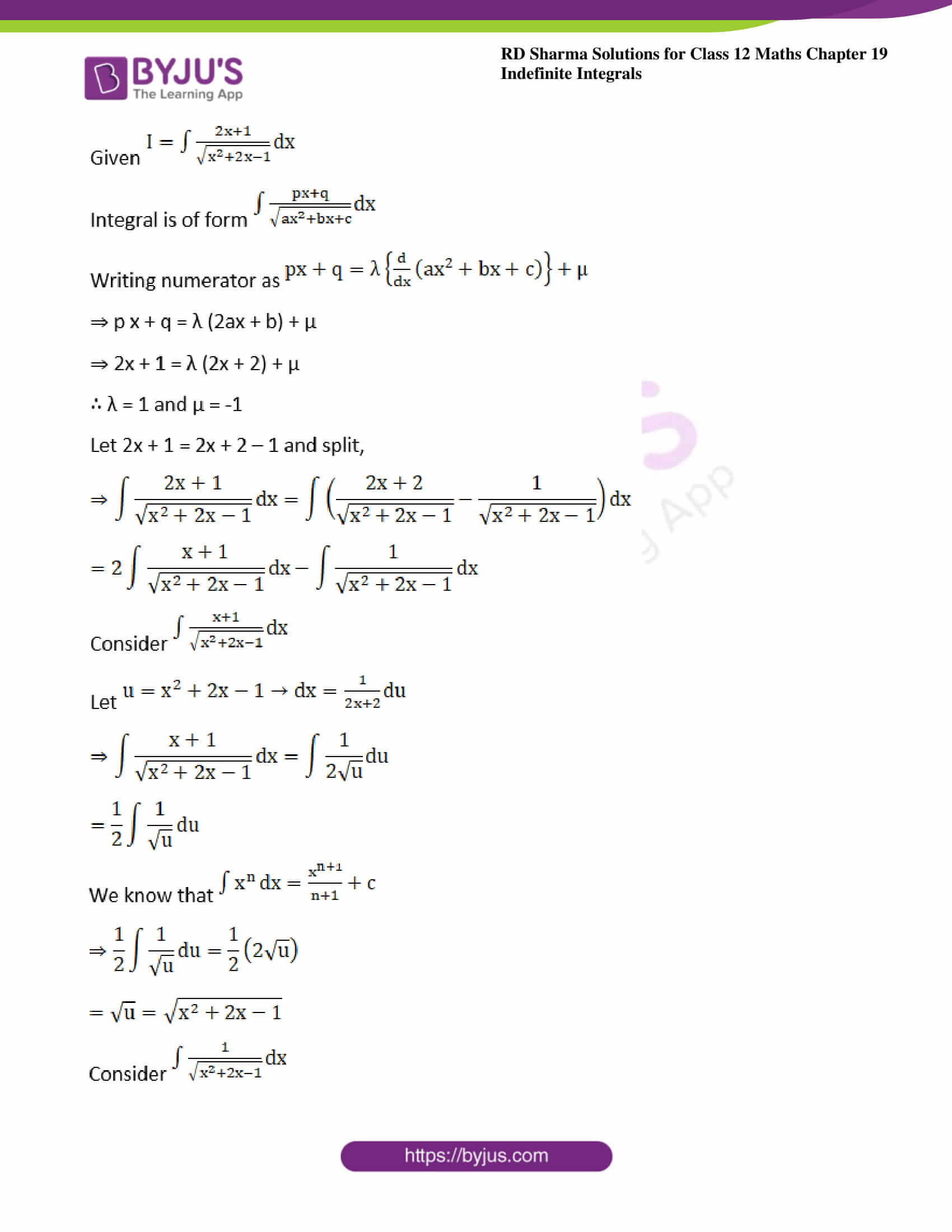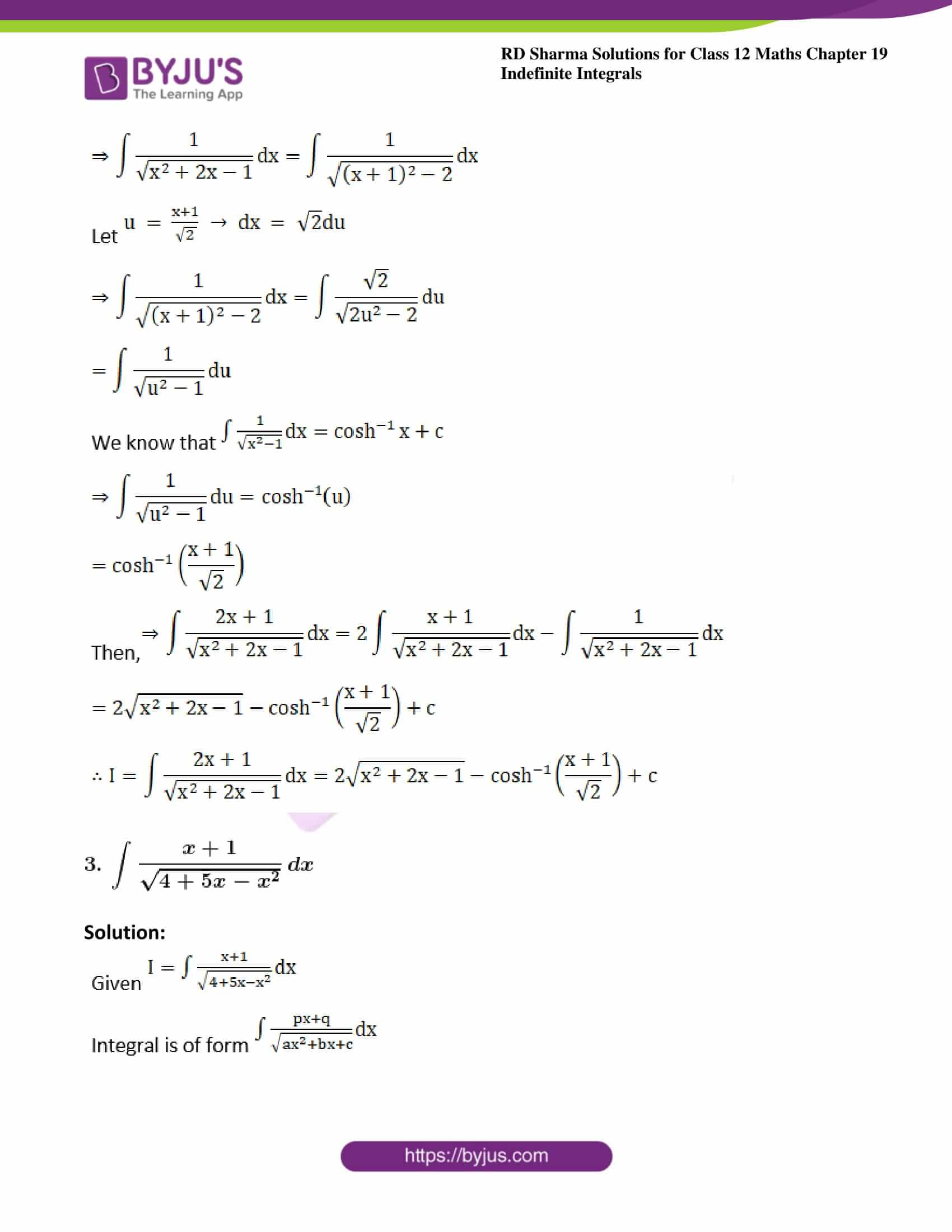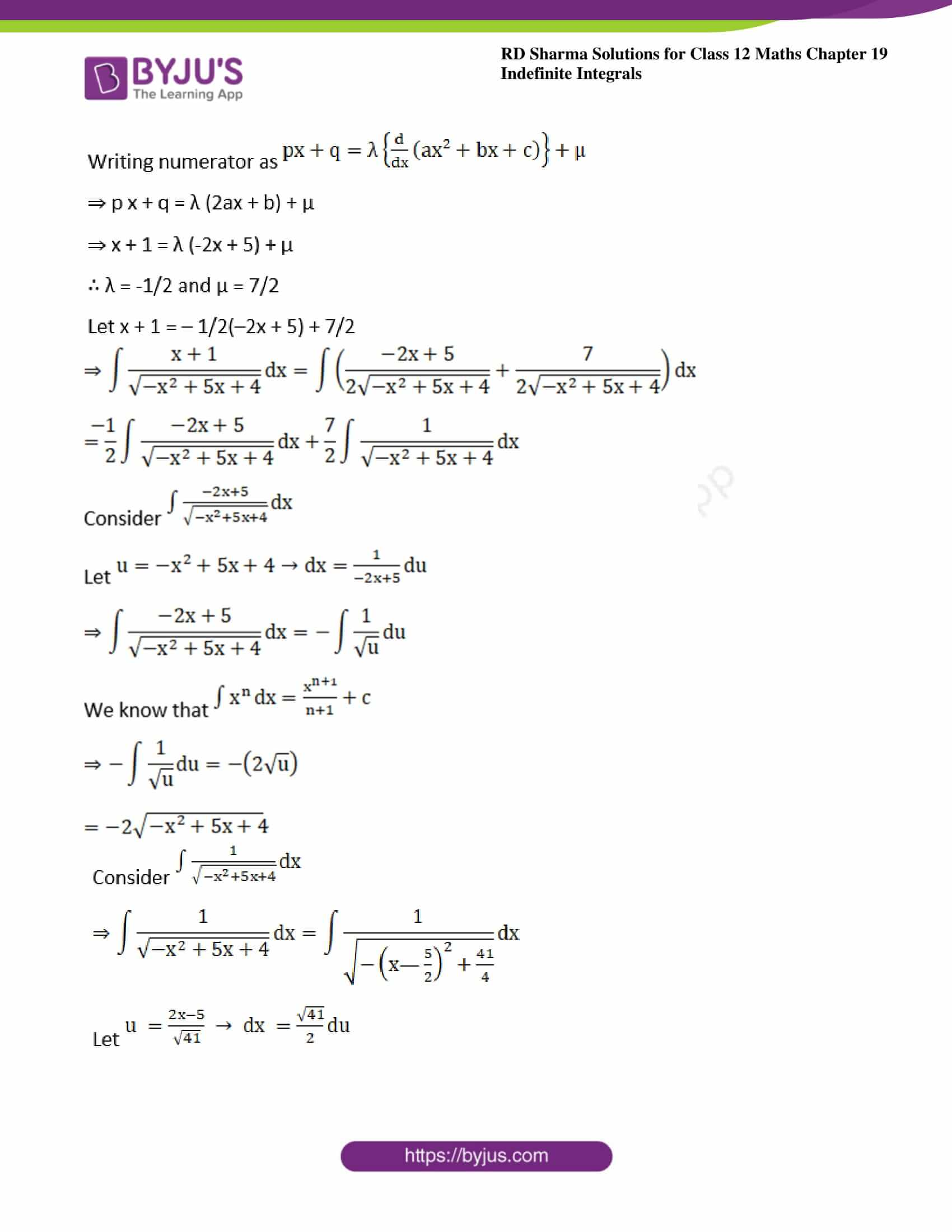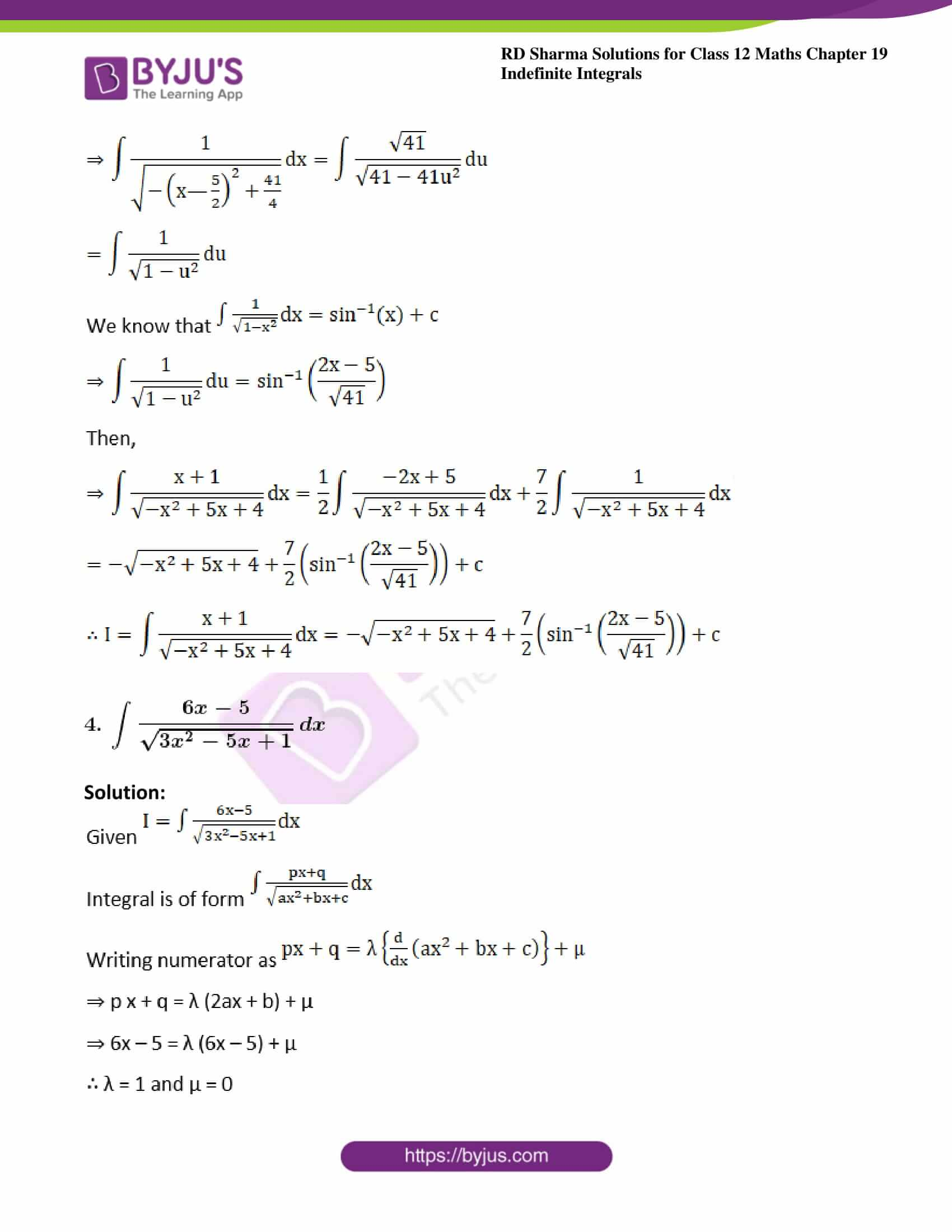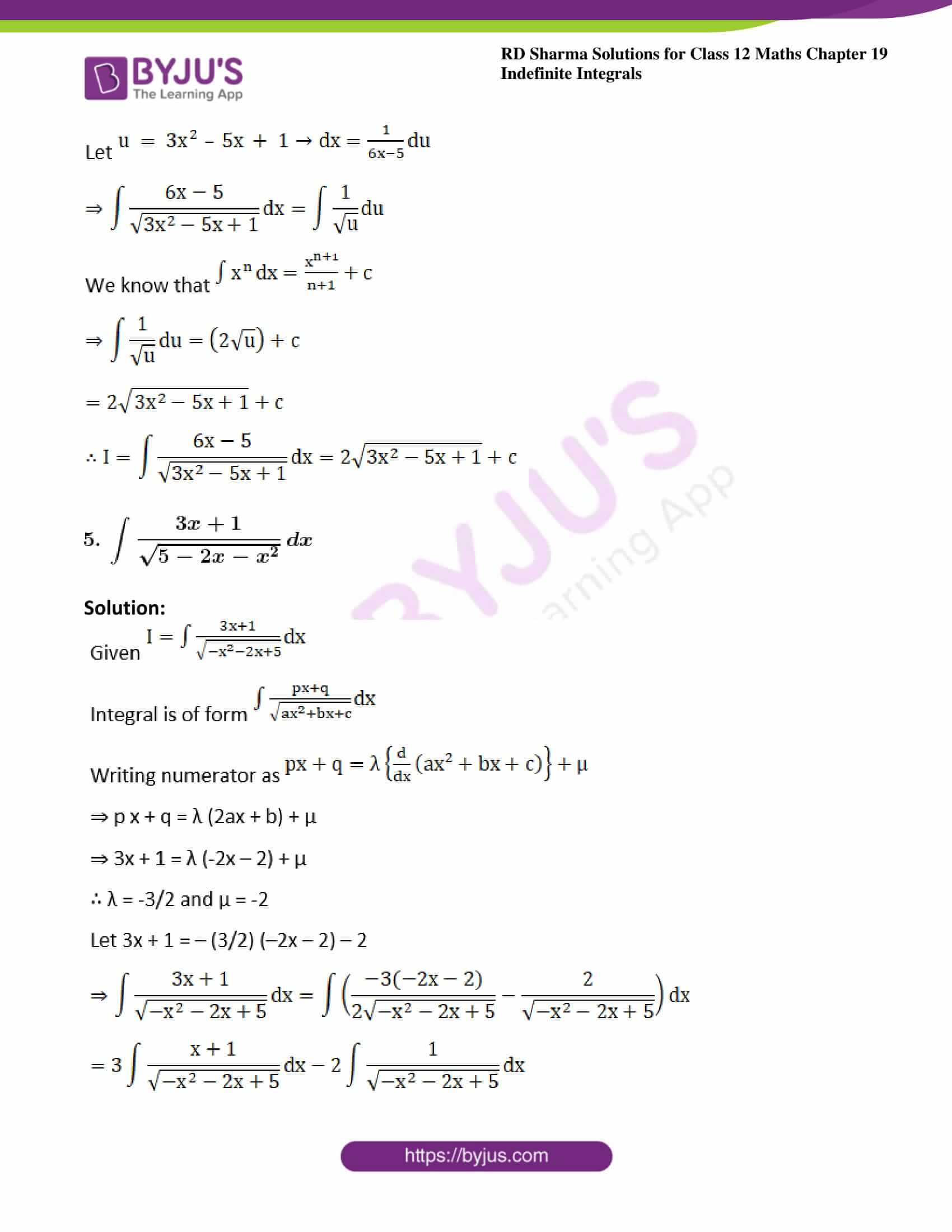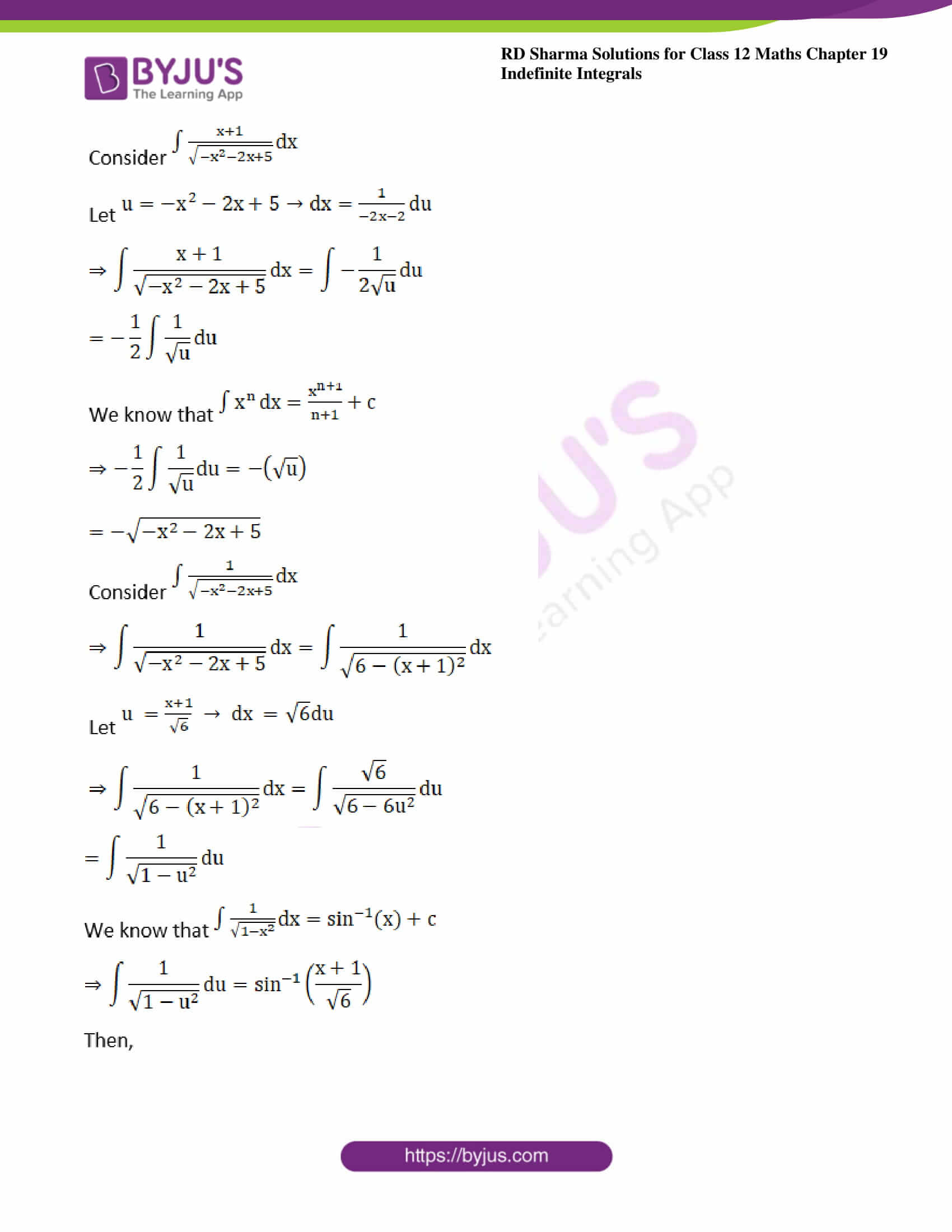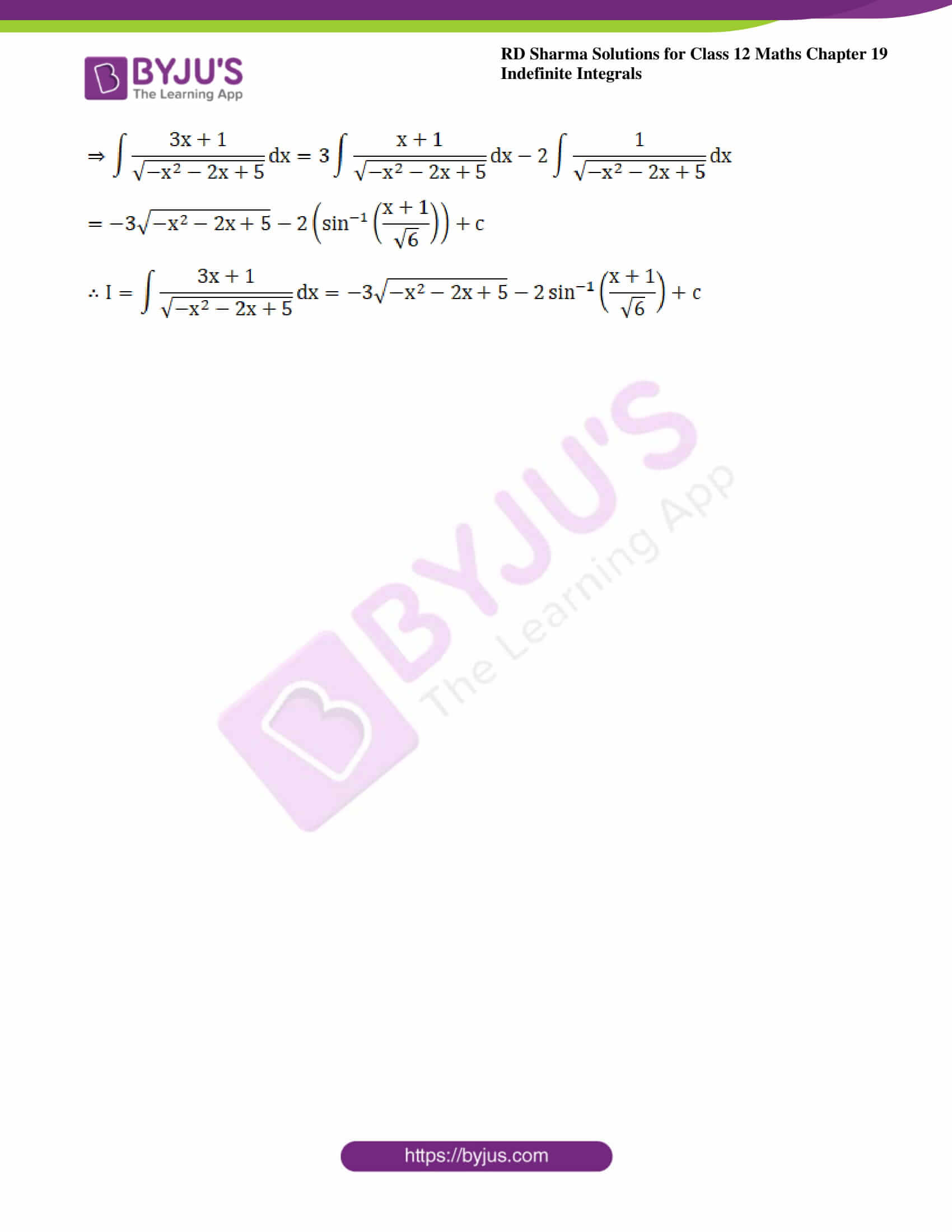### Exercise 19.21 Page No: 19.110

Evaluate the following integrals: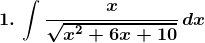Solution: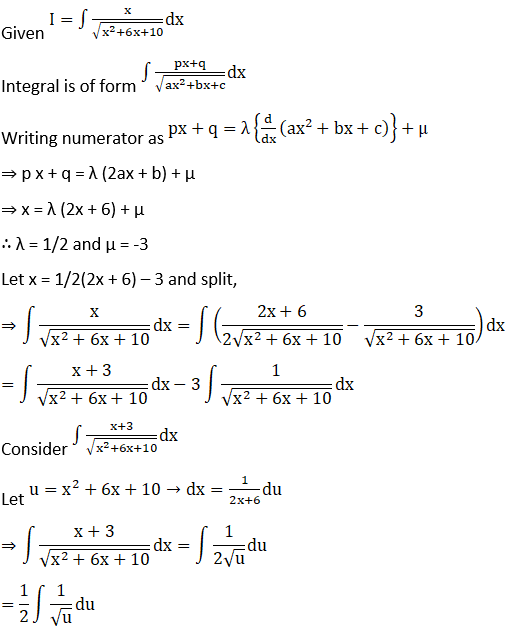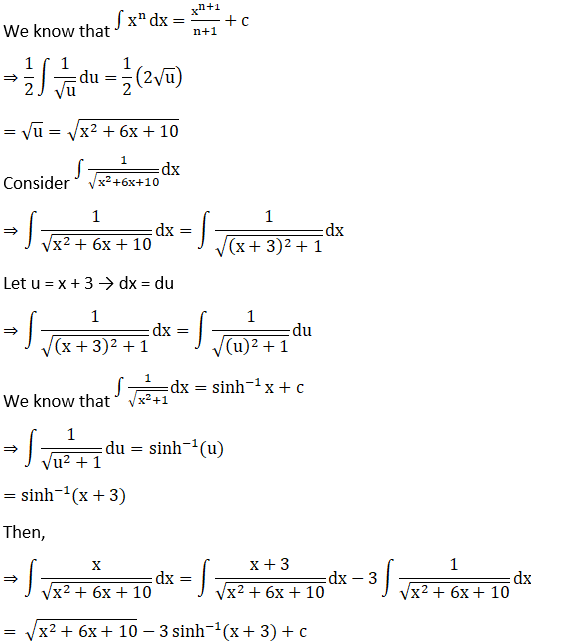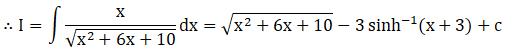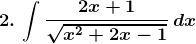Solution: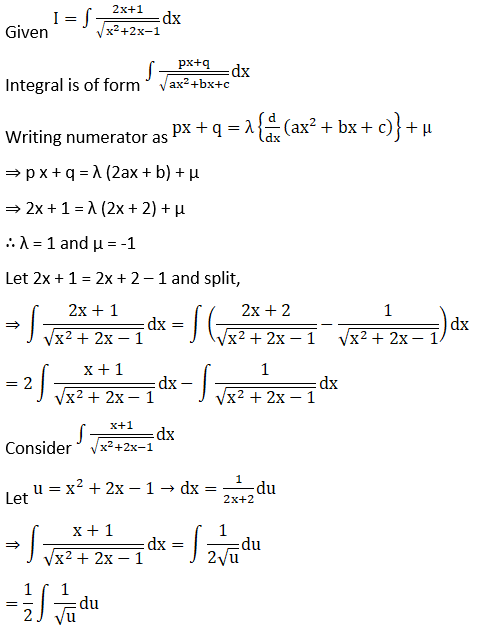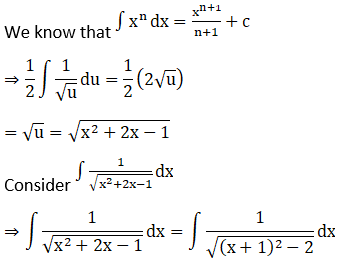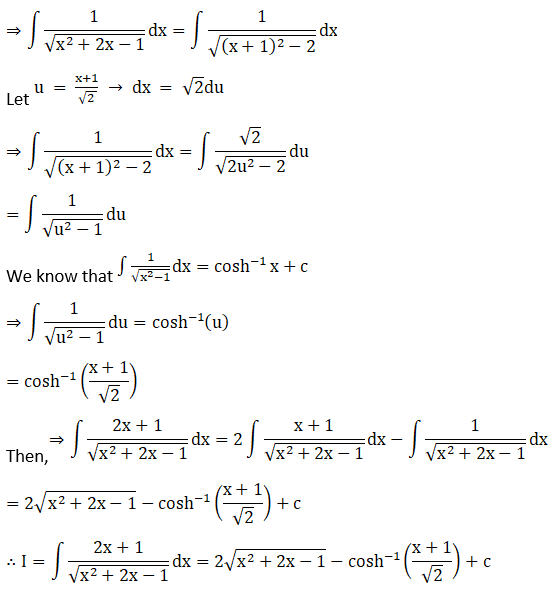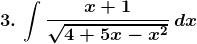Solution: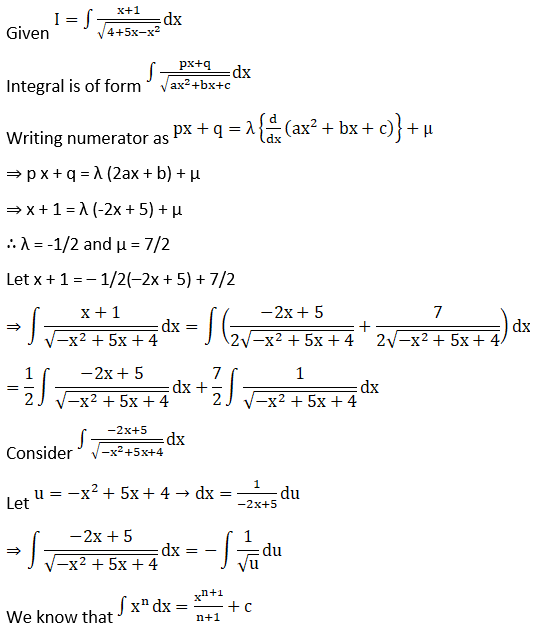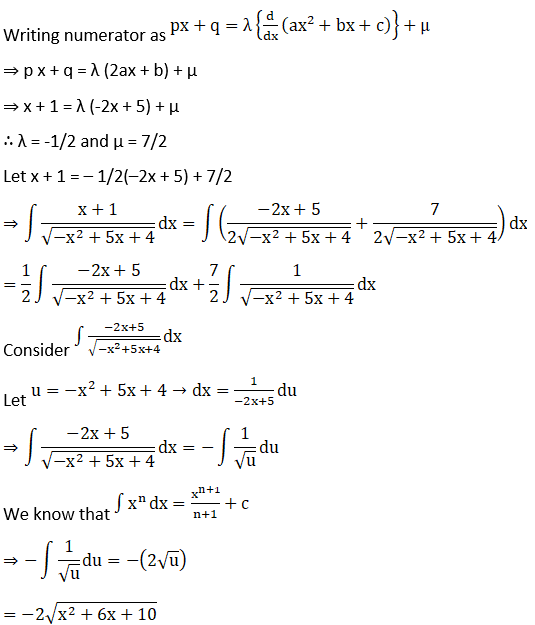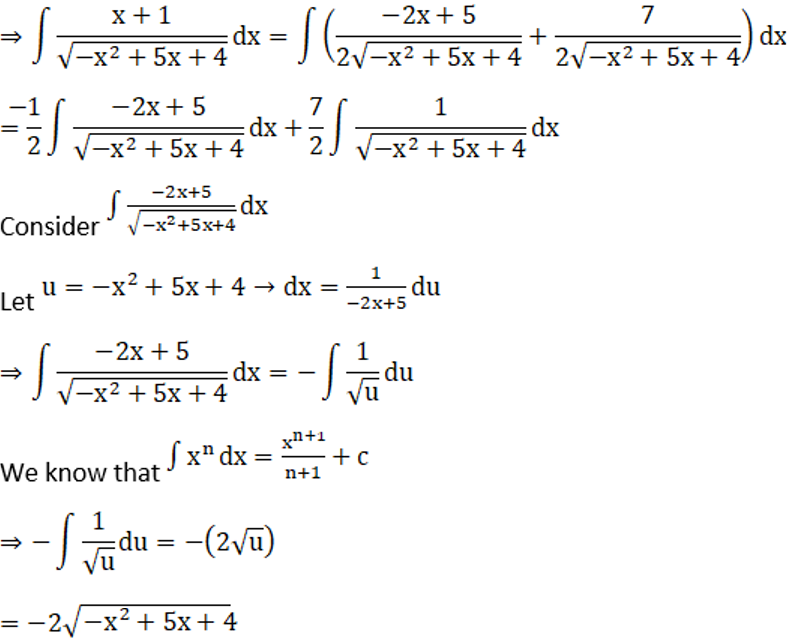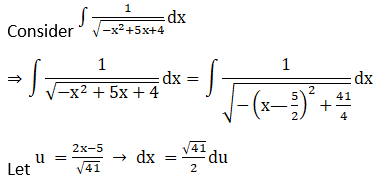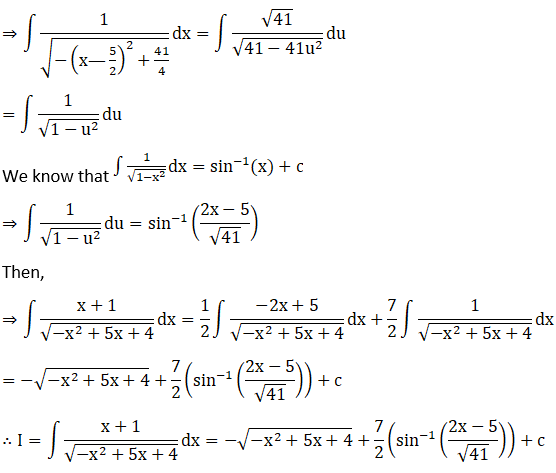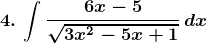Solution: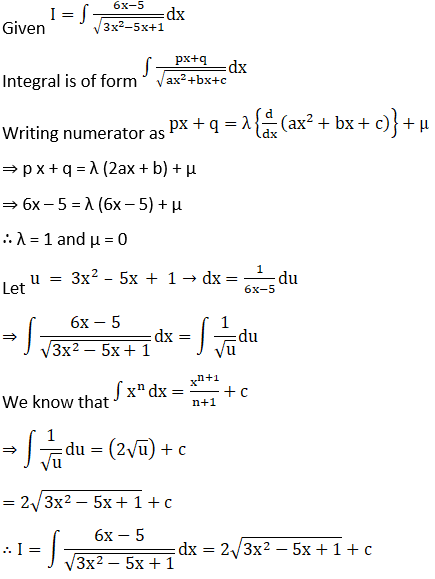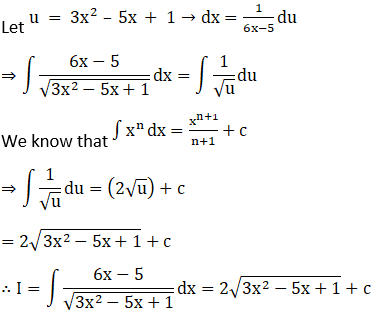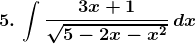Solution: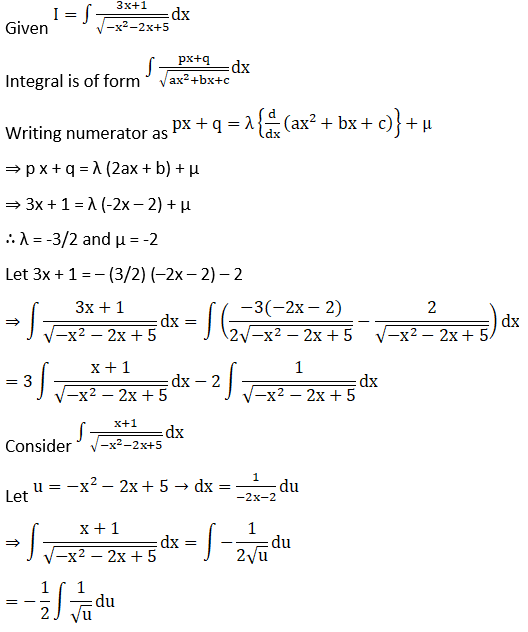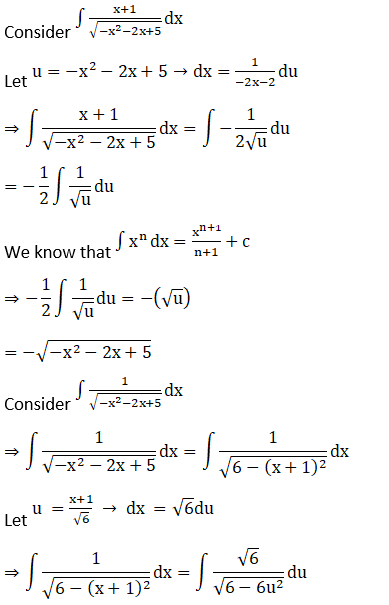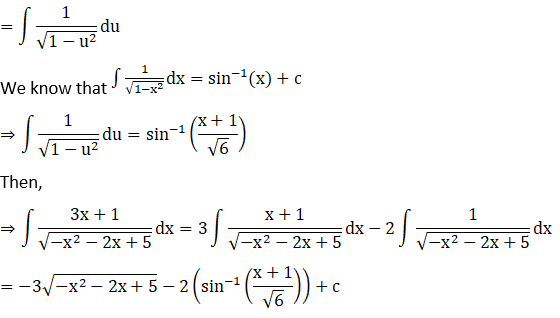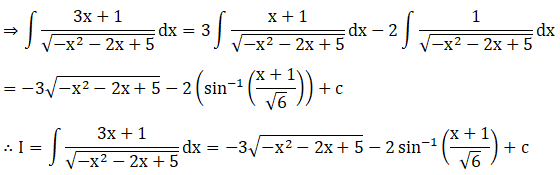### Access other exercises of RD Sharma Solutions For Class 12 Chapter 19 – Indefinite Integrals

Exercise 19.1 Solutions

Exercise 19.2 Solutions

Exercise 19.3 Solutions

Exercise 19.4 Solutions

Exercise 19.5 Solutions

Exercise 19.6 Solutions

Exercise 19.7 Solutions

Exercise 19.8 Solutions

Exercise 19.9 Solutions

Exercise 19.10 Solutions

Exercise 19.11 Solutions

Exercise 19.12 Solutions

Exercise 19.13 Solutions

Exercise 19.14 Solutions

Exercise 19.15 Solutions

Exercise 19.16 Solutions

Exercise 19.17 Solutions

Exercise 19.18 Solutions

Exercise 19.19 Solutions

Exercise 19.20 Solutions

Exercise 19.22 Solutions

Exercise 19.23 Solutions

Exercise 19.24 Solutions

Exercise 19.25 Solutions

Exercise 19.26 Solutions

Exercise 19.27 Solutions

Exercise 19.28 Solutions

Exercise 19.29 Solutions

Exercise 19.30 Solutions

Exercise 19.31 Solutions

Exercise 19.32 Solutions